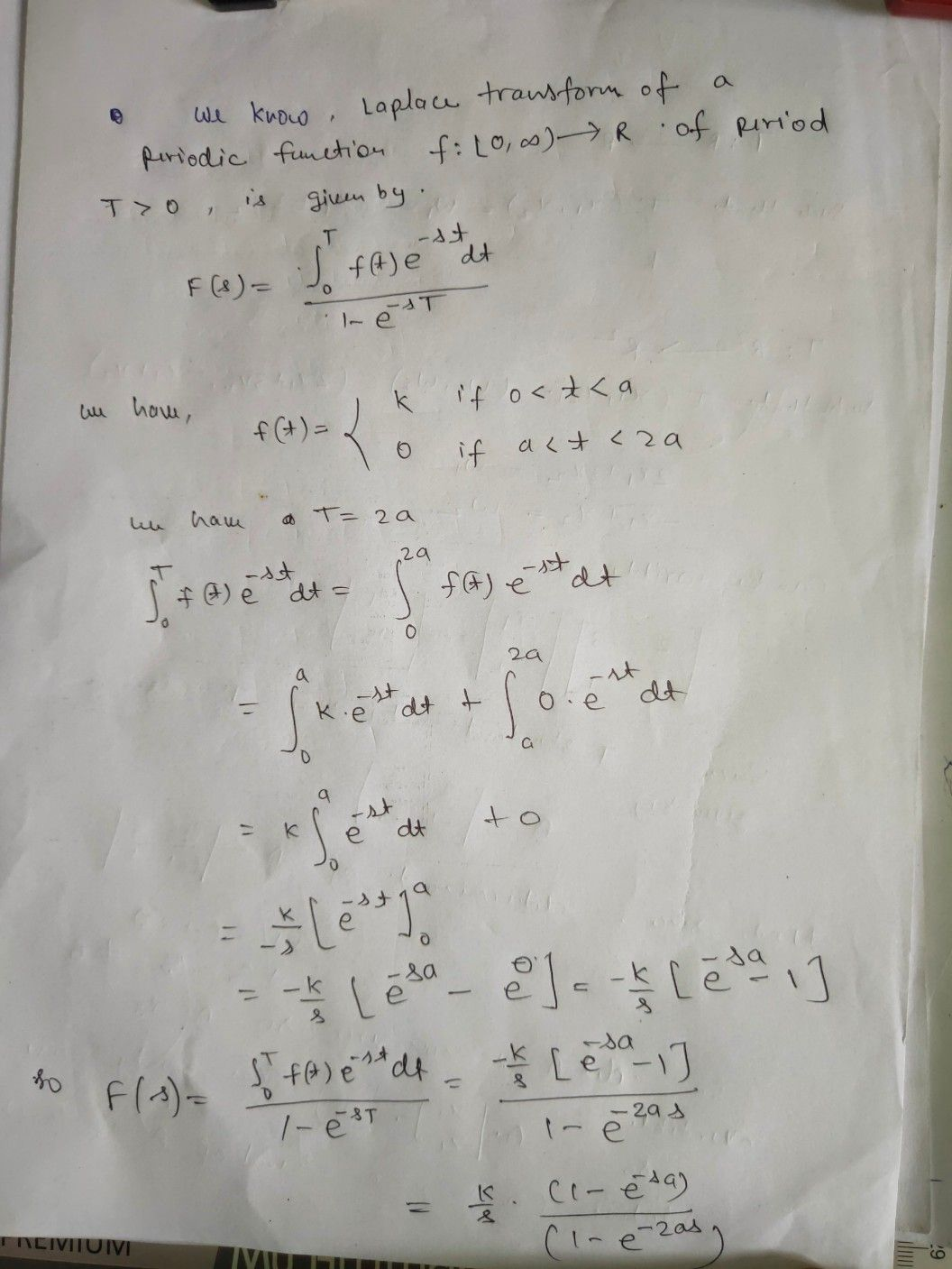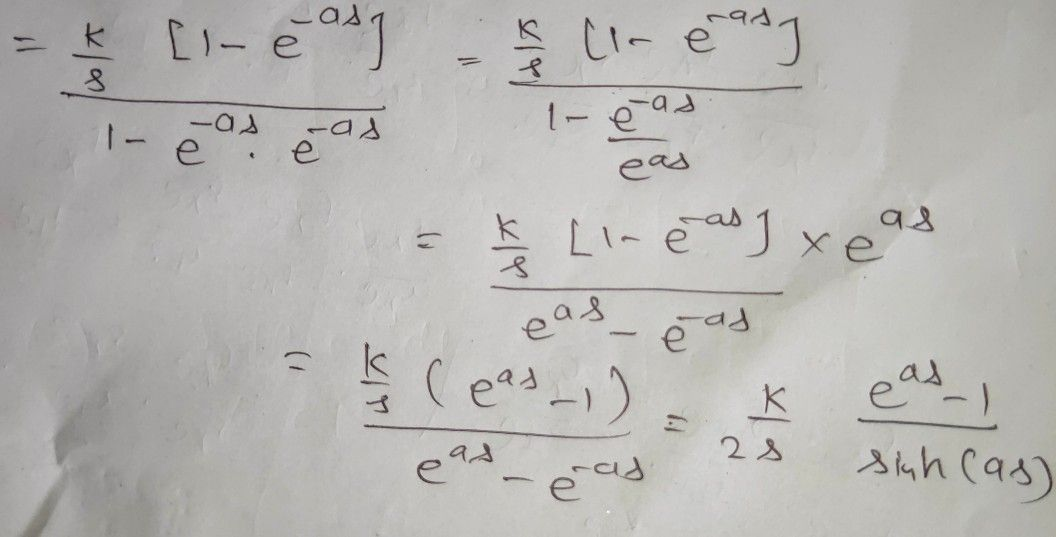Symbol
Problem$2.$ Find the Laplace transform of the function of period $2a$ defined $aS$ $f\left(t\right)= \begin{cases} k, \\ 0, \end{cases}$ $f0<t<a$ $fa<t<2a$
Calculus
Search count: 110
SolutionQanda teacher - madhusudan